Know more about the Repeaters Batch Know more about the Repeaters Batch

# Charging And Discharging Of Capacitor

A capacitor is one of several kinds of devices used in the electric circuits of radios, computers and other such equipment’s. Capacitors provide temporary storage of energy in circuits and can be made to release it when required. The property of a capacitor that characterises its ability to store energy is called its capacitance.

When energy is stored in a capacitor, an electric field exists within the capacitor. The stored energy can be associated with the electric field. Indeed, energy can be associated with the existence of an electric field. The study of capacitors and capacitance leads us to an important aspect of electric fields, the energy of an electric field.

### Table of Content

The study of capacitors and capacitance also provides the background for learning about some of the properties of insulators. Because of their behaviour in electric fields, insulators are often referred to as dielectrics.

In this lesson, we will use the concept of electric potential to examine the capacitor. Later on, we will consider polarization, in which the imposition of an electric field on a dielectric causes a net separation of charges. We shall then talk about the most important practical consequence of polarization: the way the presence of a dielectric affects the properties of a capacitor.

## Capacitance

If we go on pouring a liquid into a vessel, the level of the liquid goes on rising. Similarly, if we go on giving charge to a conductor, its potential keeps on rising. Thus:

Charge (Q) ∝ potential (V)

Or

Q = CV … (1)

Here, C is a constant of proportionality and is called the capacitance or capacity of the conductor.

From equation …(1),

C = Q / V … (2)

The capacitance of a conductor is thus defined as the ratio of the charge on it to its potential.

The value of C depends upon:

• The size and shape of the conductor,
• The nature of the medium surrounding the conductor and
• The position of the neighbouring charges.

It does not, however, depend upon the material of the conductor.

Further, let V = 1, Therefore from Eqn. (1),

Q = C or C = Q.

The capacitance of a conductor is thus numerically equal to the amount of charge required to raise its potential through unity.

The cgs unit of capacitance is called an esu of capacitance or a statfarad (st F).

$$\begin{array}{l}1\ \text{statfarad} =\frac{\text{1 statcoulomb}}{1\,\text{statvolt}}\end{array}$$

The capacitance of a conductor is thus said to be one statfarad if its potential rises through one statvolt when a charge of one statcoulomb is given to it. The SI unit of capacitance is called a farad (F).

From equation …(2),

$$\begin{array}{l}1\ \text{farad (F)} =\frac{\text{1 coulomb (C)}}{1\,\text{volt (V)}}\end{array}$$

Also Read: Energy Stored in a Capacitor

## Charging And Discharging Of A Capacitor Through A Resistor

Consider a circuit having a capacitance C and a resistance R which are joined in series with a battery of emf ε through a Morse key K as shown in the figure.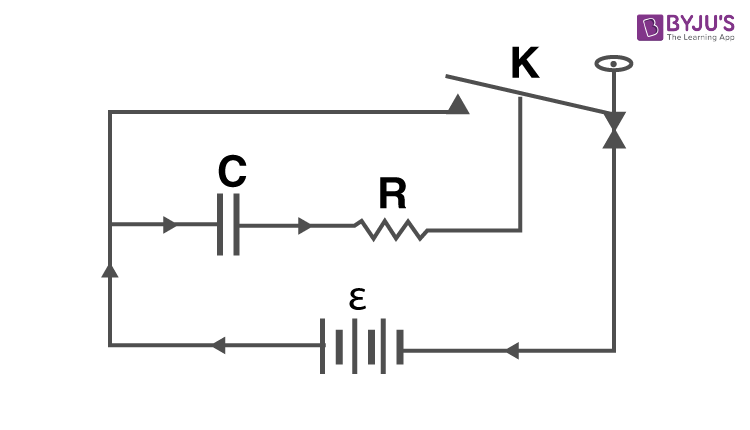### Charging of a Capacitor

When the key is pressed, the capacitor begins to store charge. If at any time during charging, I is the current through the circuit and Q is the charge on the capacitor, then

Potential difference across resistor = IR, and

Potential difference between the plates of the capacitor = Q/C

Since the sum of both these potentials is equal to ε,

RI + Q/C = ε … (1)

As the current stops flowing when the capacitor is fully charged,

When Q = Q0 (the maximum value of the charge on the capacitor), I = 0

From equation. (1),

Q0 / C = ε … (2)

From equations. (1) and (2),

$$\begin{array}{l}RI+\frac{Q}{C}=\frac{{{Q}_{0}}}{C}\end{array}$$

Or

$$\begin{array}{l}\frac{{{Q}_{0}}}{C}-\frac{Q}{C}=RI\end{array}$$

Or

$$\begin{array}{l}\frac{{{Q}_{0}}-Q}{CR}=I.…(3)\end{array}$$

Since I = dQ/dt, from equation …(3),

$$\begin{array}{l}\frac{{{Q}_{0}}-Q}{CR}=\frac{dQ}{dt}\,\,or\,\frac{dQ}{{{Q}_{0}}-Q}=\frac{dt}{CR}\end{array}$$

When t = 0, Q = 0 and when t = t, Q = Q.

Integrating both sides within proper limits, we get

$$\begin{array}{l}\int\limits_{0}^{Q}{\frac{dQ}{\left( {{Q}_{0}}-Q \right)}}=\int\limits_{0}^{t}{\frac{dt}{CR}}=\frac{1}{CR}\int\limits_{0}^{t}{dt}\end{array}$$

or

$$\begin{array}{l}\left| -\ln \left( {{Q}_{0}}-Q \right) \right|_{0}^{Q}=\frac{1}{CR}\left| t \right|_{0}^{t}\end{array}$$

or

$$\begin{array}{l}-\ln \left( {{Q}_{0}}-Q \right)+\ln {{Q}_{0}}=\frac{t}{CR}\end{array}$$

or

$$\begin{array}{l}\ln \left( {{Q}_{0}}-Q \right)-\ln {{Q}_{0}}=-\frac{t}{CR}\end{array}$$

or

$$\begin{array}{l}\ln \frac{{{Q}_{0}}-Q}{{{Q}_{0}}}=-\frac{t}{CR}\end{array}$$

or

$$\begin{array}{l}\frac{{{Q}_{0}}-Q}{{{Q}_{0}}}={{e}^{-t/CR}}\end{array}$$

or

$$\begin{array}{l}{{Q}_{0}}-Q={{Q}_{0}}{{e}^{-t/CR}}\end{array}$$

or

$$\begin{array}{l}Q={{Q}_{0}}\left( 1-{{e}^{-t/CR}} \right)\end{array}$$

or

$$\begin{array}{l}Q={{Q}_{0}}\left( 1-{{e}^{-t/\tau }} \right)…. (4)\end{array}$$

Where

$$\begin{array}{l}\tau =CR\end{array}$$

Eqn. (4) gives us the value of charge on the capacitor at any time during charging.

#### Capacitor Charging Uncharged One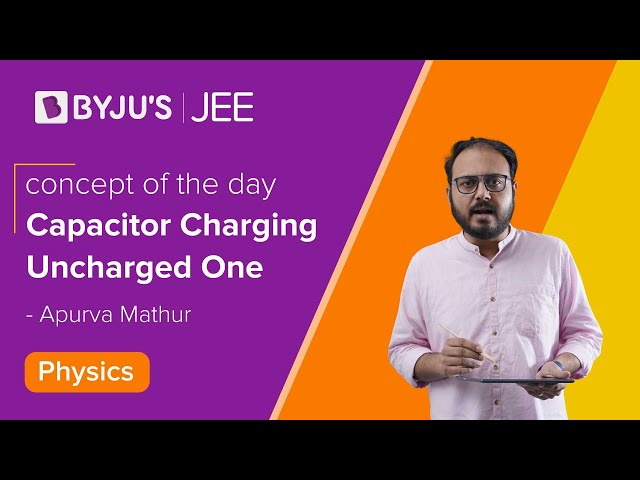### Time Constant

The dimensions of CR are those of time. Further, if CR < < 1, Q will attain its final value rapidly and if CR > > 1, it will do so slowly. Thus, CR determines the rate at which the capacitor charges (or discharges) itself through a resistance. It is for this reason that the quantity CR is called the time constant or more appropriately, the capacitive time constant of the circuit.

If t = τ, then from eqn. (4),

$$\begin{array}{l}Q={{Q}_{0}}\left( 1-{{e}^{-1}} \right)={{Q}_{0}}\left( 1-\frac{1}{e} \right)\end{array}$$

or

$$\begin{array}{l}Q={{Q}_{0}}\left( 1-\frac{1}{2.718} \right)\end{array}$$
$$\begin{array}{l}={{Q}_{0}}\left( 1-0.368 \right) = 0.632{{Q}_{0}}\end{array}$$

= 63.2% of Q0

Time constant of a CR circuit is thus the time during which the charge on the capacitor becomes 0.632 (approx., 2/3) of its maximum value.

For the charge on the capacitor to attain its maximum value (Q0), i.e., for Q = Q0,

$$\begin{array}{l}{{e}^{-t/CR}}=0\,\,\,or\,\,t=\infty\end{array}$$

Thus, theoretically, the charge on the capacitor will attain its maximum value only after infinite time.

### Discharging of a Capacitor

When the key K is released [Figure], the circuit is broken without introducing any additional resistance. The battery is now out of the circuit and the capacitor will discharge itself through R. If I is the current at any time during discharge, then putting ε = 0 in RI + Q/C = ε, we get

$$\begin{array}{l}RI+\frac{Q}{C}=0\,\,\,or\,\,\,R\frac{dQ}{dt}+\frac{Q}{C}=0\end{array}$$

or

$$\begin{array}{l}R\frac{dQ}{dt}=-\frac{Q}{C}\,\,or\,\,\frac{dQ}{Q}=-\frac{dt}{CR}\end{array}$$

When t = 0, Q = Q0 and when t = t, Q = Q.

Integrating both sides within proper limits, we get

$$\begin{array}{l}\int\limits_{{{Q}_{0}}}^{Q}{\frac{dQ}{Q}}=-\int\limits_{0}^{t}{\frac{dt}{CR}}=-\frac{1}{CR}\int\limits_{0}^{t}{dt}\end{array}$$

Or

$$\begin{array}{l}\left| \ln Q \right|_{{{Q}_{0}}}^{Q}=-\frac{1}{CR}\left| t \right|_{0}^{t}\end{array}$$

Or

$$\begin{array}{l}\ln Q-\ln {{Q}_{0}}=-\frac{t}{CR}\end{array}$$

Or

$$\begin{array}{l}\ln \frac{Q}{{{Q}_{0}}}=-\frac{t}{CR}\end{array}$$

Or

$$\begin{array}{l}Q={{Q}_{0}}{{e}^{-t/CR}}={{Q}_{0}}{{e}^{-t/\tau }}…. (5)\end{array}$$

where

$$\begin{array}{l}\tau =CR\end{array}$$

Eqn. (5) gives the value of the charge on the capacitor at any time during discharging.

If t = CR, then from eqn. (5),

$$\begin{array}{l}Q={{Q}_{0}}{{e}^{-1}}={{Q}_{0}}/e=0.368Q=36.8\%\,\,of\,\,{{Q}_{0}}\end{array}$$

Time constant of a CR circuit is thus also the time during which the charge on the capacitor falls from its maximum value to 0.368 (approx… 1/3) of its maximum value.

Thus, the charge on the capacitor will become zero only after infinite time.

The discharging of a capacitor has been shown in the figure.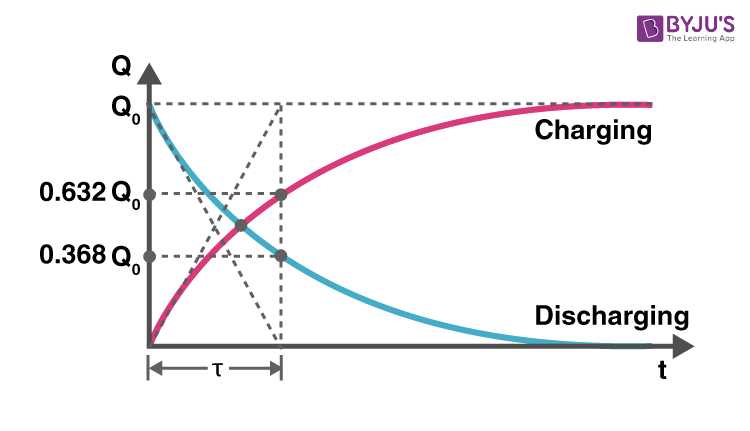## Current During Charging and Discharging of a Capacitor

We know that

$$\begin{array}{l}I=\frac{dQ}{dt}\end{array}$$

During charging:

As,

$$\begin{array}{l}Q={{Q}_{0}}\left( 1-{{e}^{-t/\tau }} \right)\end{array}$$
$$\begin{array}{l}I=\frac{d}{dt}\left( Q \right)=\frac{d}{dt}\left[ {{Q}_{0}}\left( 1-{{e}^{-t/\tau }} \right) \right]\end{array}$$

Or

$$\begin{array}{l}{{I}_{ch}}=\frac{{{Q}_{0}}}{\tau }{{e}^{-t/\tau }}={{I}_{0}}{{e}^{-t/\tau }}.… (6)\end{array}$$

Where

$$\begin{array}{l}{{I}_{0}}=\frac{{{Q}_{0}}}{\tau }=\text{maximum value of the current flowing through the circuit.}\end{array}$$

When,

$$\begin{array}{l}t=0,\,{{I}_{ch}}={{I}_{0}}\end{array}$$

During discharging:

As,

$$\begin{array}{l}Q={{Q}_{0}}{{e}^{-t/\tau }}\end{array}$$
,

$$\begin{array}{l}I=\frac{d}{dt}\left( Q \right)=\frac{d}{dt}\left( {{Q}_{0}}{{e}^{-t/\tau }} \right)\end{array}$$

Or

$$\begin{array}{l}{{I}_{dis}}=-\frac{{{Q}_{0}}}{\tau }{{e}^{-t/\tau }}=-{{I}_{0}}{{e}^{-t/\tau }}…. (7)\end{array}$$

When,

$$\begin{array}{l}t=0,\,\,{{I}_{dis}}=-{{I}_{0}}={{I}_{0}}\end{array}$$
(in magnitude)

It is clear from equations (6) and (7) that the magnitudes of the maximum values of the currents (Ich and Idis) flowing through the circuit in both the cases (charging and discharging) are the same.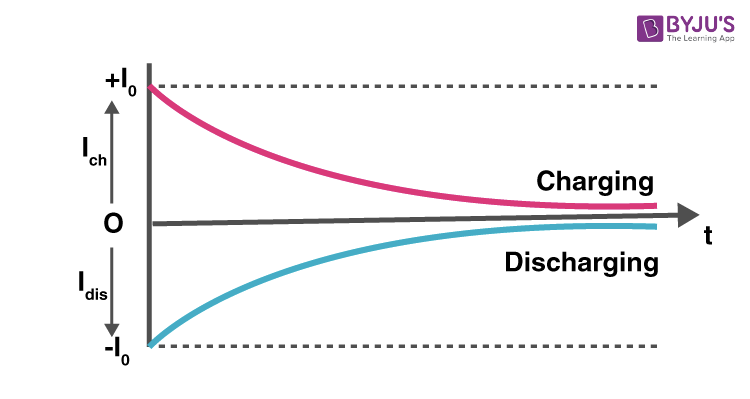Thus, both during charging and discharging of a capacitor through a resistance, the current always decreases from maximum to zero

Further, as at t = 0, Ich = I0 and Idis = -I0, the directions of flow of currents in both the cases are opposite to each other.

The change of current with time in both cases has been shown in the figure.

### Top 10 Important Questions of Electrostatic Potential and Capacitance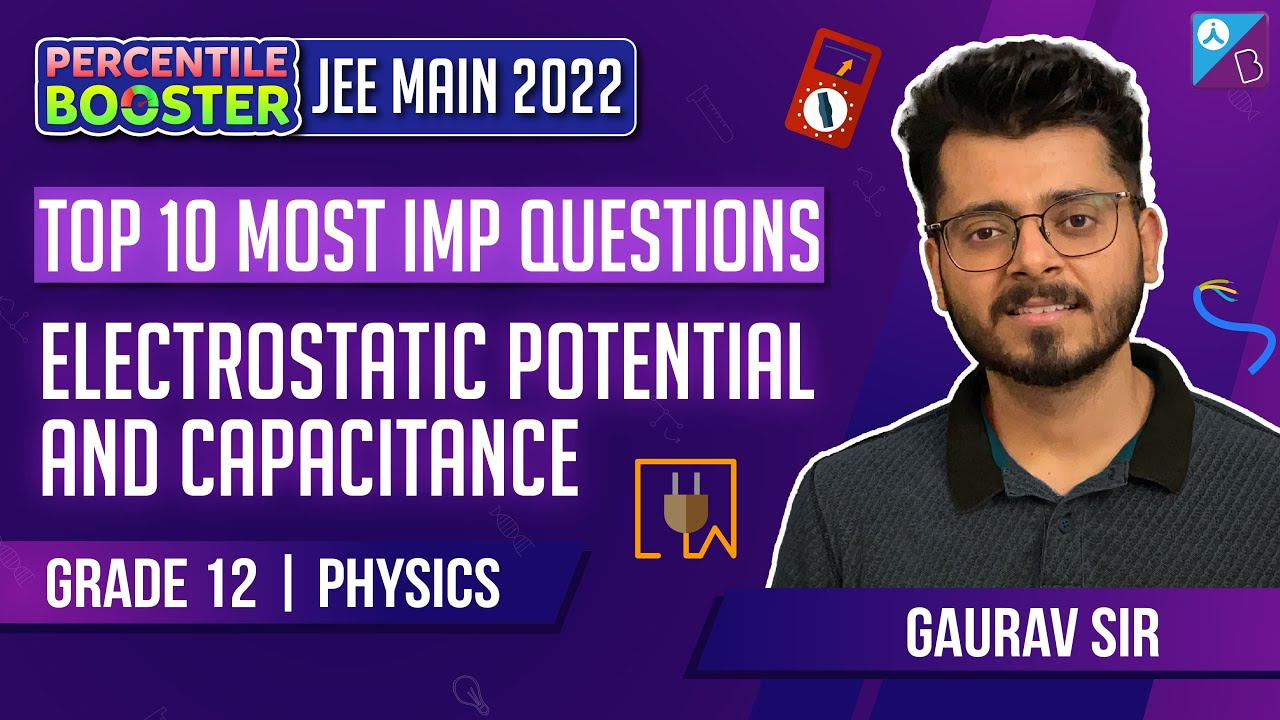## Frequently Asked Questions on Charging and Discharging of a Capacitor

### What is discharging of a capacitor?

The charge stored within the capacitor is released during discharging.

### What is the role of a dielectric in a capacitor?

When a dielectric is placed between the two conducting plates of the capacitor, it will decrease the effective potential on the two plates and hence the capacitance of the capacitor increases.

### What is the form of energy that gets stored in a capacitor?

Electric potential energy is stored in a capacitor.

### What does the rate of charging and discharging of a capacitor depend upon?

The rate of charging and discharging of a capacitor depends upon the capacitance of the capacitor and the resistance of the circuit through which it is charged.

Test your knowledge on Charging And Discharging Of Capacitor